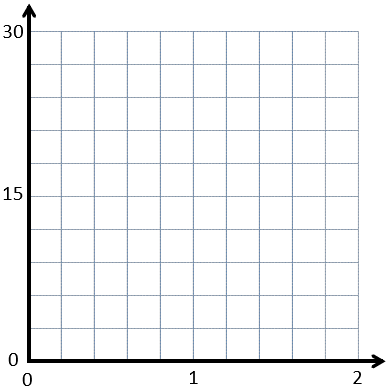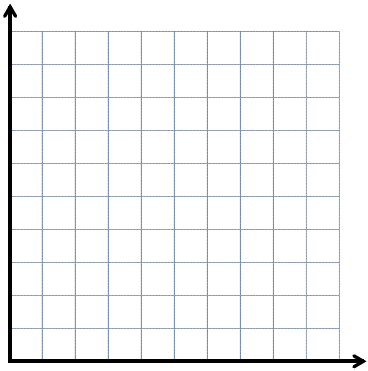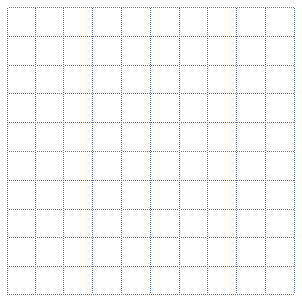# Patterns and the Coordinate Plane

## Objective

Determine the interval needed to plot various points and practice plotting and identifying points on coordinate grids with various axes.

## Common Core Standards

### Core Standards

?

• 5.G.A.1 — Use a pair of perpendicular number lines, called axes, to define a coordinate system, with the intersection of the lines (the origin) arranged to coincide with the 0 on each line and a given point in the plane located by using an ordered pair of numbers, called its coordinates. Understand that the first number indicates how far to travel from the origin in the direction of one axis, and the second number indicates how far to travel in the direction of the second axis, with the convention that the names of the two axes and the coordinates correspond (e.g., x-axis and x-coordinate, y-axis and y-coordinate).

?

• 2.MD.B.6

• 3.NF.A.2

## Criteria for Success

?

1. Decide on a scale for a coordinate graph given a set of points that must be on the graph.
2. Plot points whose x- and/or y-coordinate is/are not a multiple of the scale of the corresponding axis (i.e., the point will not be located on intersecting gridlines) (MP.6).

## Tips for Teachers

?

Similar to Lesson 3, attention to precision continues to be of great importance in Lesson 4, especially as students encounter points that are not on intersecting gridlines (MP.6).

#### Remote Learning Guidance

If you need to adapt or shorten this lesson for remote learning, we suggest prioritizing Anchor Task 2 (benefits from worked example). Find more guidance on adapting our math curriculum for remote learning here.

#### Fishtank Plus

• Problem Set
• Student Handout Editor
• Vocabulary Package

?

### Problem 1

Geraldo is plotting points on the following coordinate plane.1. Geraldo needs to plot the following four points:
• (0.8, 12)
• (1.6, 18)
• (1, 30)
• (2, 3)

Is the graph large enough to fit his points? How do you know?

1. What would be a point that he couldn't fit on the coordinate plane above? How do you know? How would the graph have to change in order for that point to fit on it?

### Problem 2

Determine the correct scale for the coordinate grid below so that all of the following points can be shown on it:

• (12, 4)
• (4, ${1\over2}$)
• (6, $3{1\over2}$)
• (12, $2{1\over2}$)
• (17, 4)
• (16, $4{1\over4}$)## Problem Set & Homework

#### Discussion of Problem Set

• What scale did you choose for the x-axis in #1? The y-axis?
• Where did you plot the point (0, $3\frac{1}{4}$) in #1? What was more difficult about plotting this point compared to the others?
• What scale did you choose for the x-axis in #2? The y-axis?
• Where did you plot the point (0.25, 7) in #2? What was more difficult about plotting this point compared to the others?
• Explain your thought process as you decided how to label the whole numbers along the x- and y-axes in #3.

?

Use the grid below to construct a coordinate grid on which you can plot the following points. Then plot and label the points.

 Point Coordinates A (0, 12) B ($\frac{1}{2}$, 3) C (4, 0)### Mastery Response

?